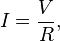## How to calculate resistors for leds

For the, what resistor should i put in the circuit so my led don’t fry question. There is a very easy way, go to a on-line calculator site like http://ledcalculator.net/, input the values and bazinga you get an instant answer.

But that’s for sissies, the only way a real man does it, is calculating (preferably by head) applying Ohm’s Law:

#####(behold, and bow twice, please)

Current (amps) equals to the proportion between potential difference (volts) and the resistance (ohms). Let’s say for a common red led (voltage is 2volts and current rating is 20mA), and a 9v battery power supply, the math is this:

(9v – 2v) / (20mA/1000A) = x Ohms
7 / 0.02 = 350 Ohms

But with these values in a led calculator, the result is 360 Ohms, what’s wrong with your math? Nothing. This is because of the so called “nearest preferred values resistors”. Resistors are available in a number of standard ranges (being the most common E24) in predefined scale. So you should round up to the next higher value. And from 350 Ohms the preferred resistor value is 360 Ohms.

With the resistance calculation done, you must find the right resistor. Again, there is the sissies method, go to a on-line resistor color code calculator like http://www.electronics2000.co.uk/calc/resistor-code-calculator.php, input the resistance and get the color codes (and vice-versa) or the mens method trough a resistor table:So, a 360 Ohms resistor is the one in your resistors packs with a Orange (3), Blue (6) and Brown (36 * 10 = 360) band. The last band, that stands for tolerance is probably gold (with my very little experience in electronics never saw a silver resistor).

Disclaimer: remember that (as always) i decline every liability if you burn your LEDs, your head or the whole world because of this post.